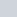# Using an Adaptive Component to measure surface area accurately

Remember Julien Benoit’s adaptive component tutorial from a year ago (link here)?  Well, Julien has made something pretty cool and posted it on RFO.  It is basically a 4 point adaptive component that calculates the area of the resulting surface using Bretschneider’s formula.

Julien’s solution (using an intense formula):AreaTool#2013_AC_CP_4P.rfa 208.0 KB

This is the formula, if you are interested (!)
sqrt((P – A) * (P – B) * (P – C) * (P – D) – ((A * B * C * D) * cos((BB + CC) / 2) ^ 2))

Alfredo’s more volumetric solution:Project_panel_areas.rvt 960.0 KB